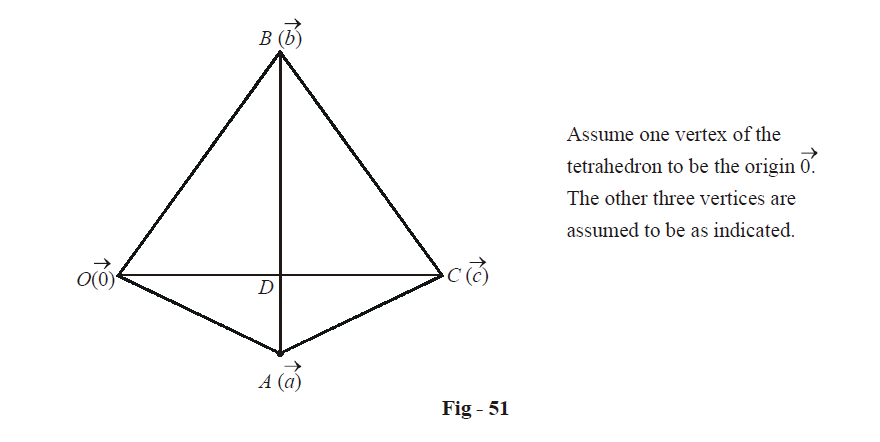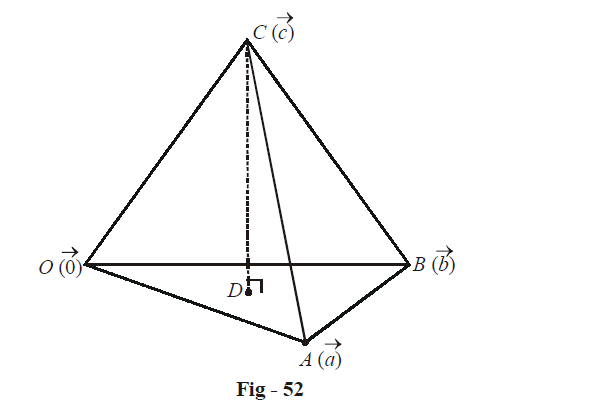# Examples On Vectors Applied To Tetrahedrons Set-1

Go back to  'Vectors and 3-D Geometry'

In the previous sections, we’ve already seen that vectors can be a powerful technique for geometrical problems, especially three dimensional ones.

In this section, we’ll study the vectors approach to geometrical problems more deeply.

For a start, let us discuss some more geometry of tetrahedrons.

Example – 40

A regular tetrahedron is one in which all edges are equal. Prove that any two opposite edges in a regular tetrahedron are perpendicular.

Solution:Since all the edges of this tetrahedron are equal, we have

$\left| {\vec a} \right| = \left| {\vec b} \right| = \left| {\vec c} \right| = \left| {\vec a - \vec b} \right| = \left| {\vec b - \vec c} \right| = \left| {\vec c - \vec a} \right|$

We need to prove that any two opposite edges are perpendicular. Lets prove that  $$\overrightarrow {OC}$$ is perpendicular to $$\overrightarrow {AB} .$$ The other cases can be proved similarly.

Since

$\left| {\vec a - \vec b} \right| = \left| {\vec a - \vec c} \right|$

we have,

\begin{align}&\qquad\;\; {\left| {\vec a - \vec b} \right|^2} = {\left| {\vec a - \vec c} \right|^2} \hfill \\\\& \Rightarrow \quad {\left| {\vec a} \right|^2} + {\left| {\vec b} \right|^2} - 2\vec a \cdot \vec b = {\left| {\vec a} \right|^2} + {\left| {\vec c} \right|^2} - 2\vec a \cdot \vec c\qquad\qquad\qquad...\left( 1 \right) \hfill \\ \end{align}

Since  $$\left| {\vec a} \right| = \left| {\vec b} \right| = \left| {\vec c} \right|,$$ (1) reduces to

$\vec a \cdot \vec b = \vec a \cdot \vec c$

Similarly,

$\vec b \cdot \vec c = \vec a \cdot \vec c\qquad\qquad\qquad...\left( 2 \right)$

(2) upon rearrangement gives

\begin{align}&\qquad \left( {\vec a - \vec b} \right) \cdot \vec c = 0 \hfill \\&\Rightarrow \quad \overrightarrow {BA} \cdot \overrightarrow {OC} = 0 \hfill \\&\Rightarrow \quad \overrightarrow {BA} \,\,is{\text{ }}perpendicular{\text{ }}to\,\,\overrightarrow {OC} {\text{ }}. \hfill \\ \end{align}

This completes our proof.

Example – 41

Let $$\vec a,\,\vec b,\,\vec c$$ represent three co-initial edges of a tetrahedron. Prove that the volume V of the tetrahedron is given by \begin{align}\vec V = \frac{1}{6}\left[ {\vec a\,\,\vec b\,\,\vec c} \right].\end{align}

Solution: Let one vertex of the tetrahedron be the origin $$O(\vec 0)$$ while the other three vertices be $$A(\vec a),\,\,B(\vec b)$$ and $$C(\vec c),$$ as shown:We will use the geometrical fact that the volume of a tetrahedron equals \begin{align}\frac{1}{3}\end{align}× (Area of base) × (height).

Let us evaluate the height of the tetrahedron DC from C to the triangular face OAB.

A unit vector $$\hat n,$$ along DC, is given by

$\hat n = \frac{{\vec a \times \vec b}}{{\left| {\vec a \times \vec b} \right|}}$

The height CD can be obtained as the projection of OC onto the line along CD:

\begin{align}&\;CD = \;\overrightarrow {OC} \cdot \hat n \hfill \\\\&\qquad = \frac{{\left( {\vec a \times \vec b} \right) \cdot \vec c}}{{\left| {\vec a \times \vec b} \right|}} \hfill \\\\&\qquad = \frac{{\left[ {\vec a\,\,\vec b\,\,\vec c} \right]}}{{\left| {\vec a \times \vec b} \right|}} \hfill \\ \end{align}

Thus, the volume V is

\begin{align} &\;V\; = \frac{1}{3} \times ({\text{area of }}\Delta OAB) \times CD \hfill \\&\quad\; = \frac{1}{3} \times \left( {\frac{1}{2}\left| {\vec a \times \vec b} \right|} \right) \times \frac{{\left[ {\vec a\,\,\vec b\,\,\vec c} \right]}}{{\left| {\vec a \times \vec b} \right|}} \hfill \\&\quad\; = \frac{1}{6}\left[ {\vec a\,\,\vec b\,\,\vec c} \right] \hfill \\ \end{align}

Vectors
grade 11 | Questions Set 1
Vectors